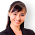## Search This Blog

This website completely moved to new domain. For latest content, visit www.programmingposts.com

### Implementing Addition of Two Matrices Using 2D-Arrays:

```using System;
namespace MatrixAdditionProgram
{
class Program
{
static void Main(string[] args)
{
//using 2D-ARRAYS
int RowSize = 5, ColSize = 5;
int[,] Matrix1 = new int[RowSize, ColSize];
int[,] Matrix2 = new int[RowSize, ColSize];
int[,] ResultMatrix = new int[RowSize, ColSize];
int i, j;

try
{
Console.WriteLine("\n >>> PROGRAM To PRINT ADDITION OF TWO MATRICES <<<");
Console.Write("\n Enter the Size of a Matrix N*N (For Example:3*3) : ");
string s = Console.ReadLine();
string[] values = s.Split('*');
RowSize = int.Parse(values);
ColSize = int.Parse(values);

if (RowSize > 5 || ColSize > 5)   //limiting the size of matrix
{
Console.BackgroundColor = ConsoleColor.DarkRed; //changing background color to red
Console.WriteLine(" The Size Of Matrix should Be in Less Than 5 (limiting size of array)");
System.Console.ResetColor(); ///resetting color
Console.WriteLine("\n\n\t Press Enter key to exit....");
Console.ReadKey(); return;
}

else
{

//Initializing all the elements to zero
for (i = 0; i < RowSize; i++)
{
for (j = 0; j < ColSize; j++)
{
Matrix1[i, j] = 0;
Matrix2[i, j] = 0;
}
}
//Reading elements of Matrix1
Console.WriteLine("\n Enter the elements of Matrix1({0}*{1})", RowSize, ColSize);
for (i = 0; i < RowSize; i++)
{
for (j = 0; j < ColSize; j++)
{
Console.Write(" Matrix1[{0},{1}] : ", i, j);
Matrix1[i, j] = Convert.ToInt32(Console.ReadLine());
}
}
//Reading elements of Matrix2
Console.WriteLine("\n Enter the elements of Matrix2({0}*{1})", RowSize, ColSize);
for (i = 0; i < RowSize; i++)
{
for (j = 0; j < ColSize; j++)
{
Console.Write(" Matrix2[{0},{1}] : ", i, j);
Matrix2[i, j] = Convert.ToInt32(Console.ReadLine());
}
}

//calculating ResultMatrix, by adding Matrix1 and Matrix2
for (i = 0; i < RowSize; i++)
{
for (j = 0; j < ColSize; j++)
{
ResultMatrix[i, j] = Matrix1[i, j] + Matrix2[i, j];
}
}

//Printing Result Matrix
Console.Write("\n\n\t*** Result Matrix  ***\n\n\t");
for (i = 0; i < RowSize; i++)
{

for (j = 0; j < ColSize; j++)
{
if (ResultMatrix[i, j] < 10)
{
Console.Write("  0" + Convert.ToString(ResultMatrix[i, j])); //Making number as 01,02,etc,.
}
else
{
Console.Write("  " + Convert.ToString(ResultMatrix[i, j]));
}

if (j == ColSize - 1) { Console.Write("\n\t"); }

}
}
}
}
catch  //to catch exceptions,suppose string entered as aRowSize or Colsize  of matrix
{
Console.BackgroundColor = ConsoleColor.DarkRed;
Console.WriteLine("WARNING:only Number are allowed, Enter Correct Input");
Console.ResetColor();
}

Console.WriteLine("\n\n\t Press Enter key to exit....");
Console.ReadLine();
}
}
}
```

In the above program the code can be shortened by reducing the number of for loops, but for making it easier to understand by the beginners, the program is made as simple as possible. Some of the different Sample Outputs of the above program are shown below:

Output1:

Output 2:

Output 3:

For C Program visit:

More Posts:

### C# PROGRAM PRINT A MULTI-DIMENSIONAL ARRAY IN SNAIL SHELL WAY

#### 6 comments:

1.Appreciation for nice Updates, I found something new and folks can get useful info about BEST ONLINE TRAINING

2.3.nice article .thank you.
web programming tutorial
welookups

4.This is good piece of writing and pleasant urging commented
here, I am really enjoying by these.
KissAnime

5.Very nice post here and thanks for it .I always like and such a super contents of these post.Excellent and very cool idea and great content of different kinds of the valuable information's.
Java training in Chennai

Java training in Bangalore

6.After reading this web site I am very satisfied simply because this site is providing comprehensive knowledge for you to audience.
Thank you to the perform as well as discuss anything incredibly important in my opinion. We loose time waiting for your next article writing in addition to I beg one to get back to pay a visit to our website in

Selenium training in bangalore
Selenium training in Chennai
Selenium training in Bangalore
Selenium training in Pune
Selenium Online training